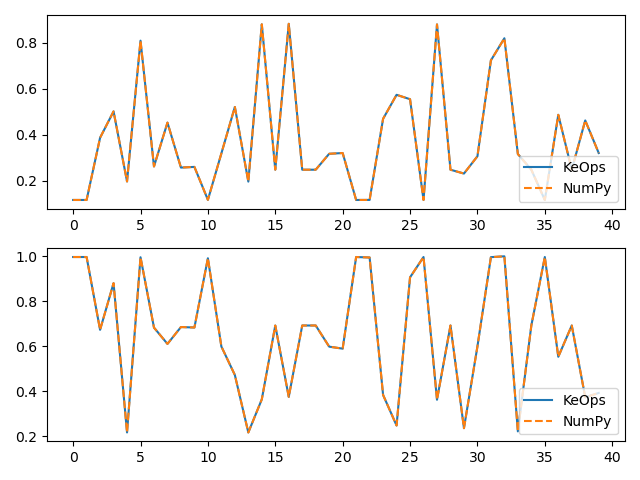# SumSoftMaxWeight reduction (with LazyTensors)

Using the pykeops.numpy.Genred API, we show how to perform a computation specified through:

• Its inputs:

• $$x$$, an array of size $$M\times 3$$ made up of $$M$$ vectors in $$\mathbb R^3$$,

• $$y$$, an array of size $$N\times 3$$ made up of $$N$$ vectors in $$\mathbb R^3$$,

• $$b$$, an array of size $$N\times 2$$ made up of $$N$$ vectors in $$\mathbb R^2$$.

• Its output:

• $$c$$, an array of size $$M\times 2$$ made up of $$M$$ vectors in $$\mathbb R^2$$ such that

$c_i = \frac{\sum_j \exp(K(x_i,y_j))\,\cdot\,b_j }{\sum_j \exp(K(x_i,y_j))},$

with $$K(x_i,y_j) = \|x_i-y_j\|^2$$.

## Setup

Standard imports:

import time

import matplotlib.pyplot as plt
import numpy as np

from pykeops.numpy import LazyTensor as kf
from pykeops.numpy import Vi, Vj
from pykeops.numpy.utils import WarmUpGpu


Define our dataset:

M = 5000  # Number of "i" points
N = 4000  # Number of "j" points
D = 3  # Dimension of the ambient space
Dv = 2  # Dimension of the vectors

x = 2 * np.random.randn(M, D)
y = 2 * np.random.randn(N, D)
b = np.random.rand(N, Dv)

# KeOps implementation with the helper
WarmUpGpu()
start = time.time()
c = kf.sum((Vi(x) - Vj(y)) ** 2, axis=2)
c = kf.sumsoftmaxweight(c, Vj(b), axis=1)
print("Timing (KeOps implementation): ", round(time.time() - start, 5), "s")

# compare with direct implementation
start = time.time()
cc = np.sum((x[:, None, :] - y[None, :, :]) ** 2, axis=2)
cc -= np.max(cc, axis=1)[:, None]  # Subtract the max to prevent numeric overflows
cc = np.exp(cc) @ b / np.sum(np.exp(cc), axis=1)[:, None]
print("Timing (Numpy implementation): ", round(time.time() - start, 5), "s")

print("Relative error : ", (np.linalg.norm(c - cc) / np.linalg.norm(c)).item())

# Plot the results next to each other:
for i in range(Dv):
plt.subplot(Dv, 1, i + 1)
plt.plot(c[:40, i], "-", label="KeOps")
plt.plot(cc[:40, i], "--", label="NumPy")
plt.legend(loc="lower right")
plt.tight_layout()
plt.show()[pyKeOps] Warming up the Gpu (numpy bindings) !!!
[pyKeOps] Warning : keyword argument dtype in Genred is deprecated ; argument is ignored.
Timing (KeOps implementation):  0.00282 s
Timing (Numpy implementation):  0.81745 s
Relative error :  5.521489980421426e-16


Total running time of the script: ( 0 minutes 0.970 seconds)

Gallery generated by Sphinx-Gallery